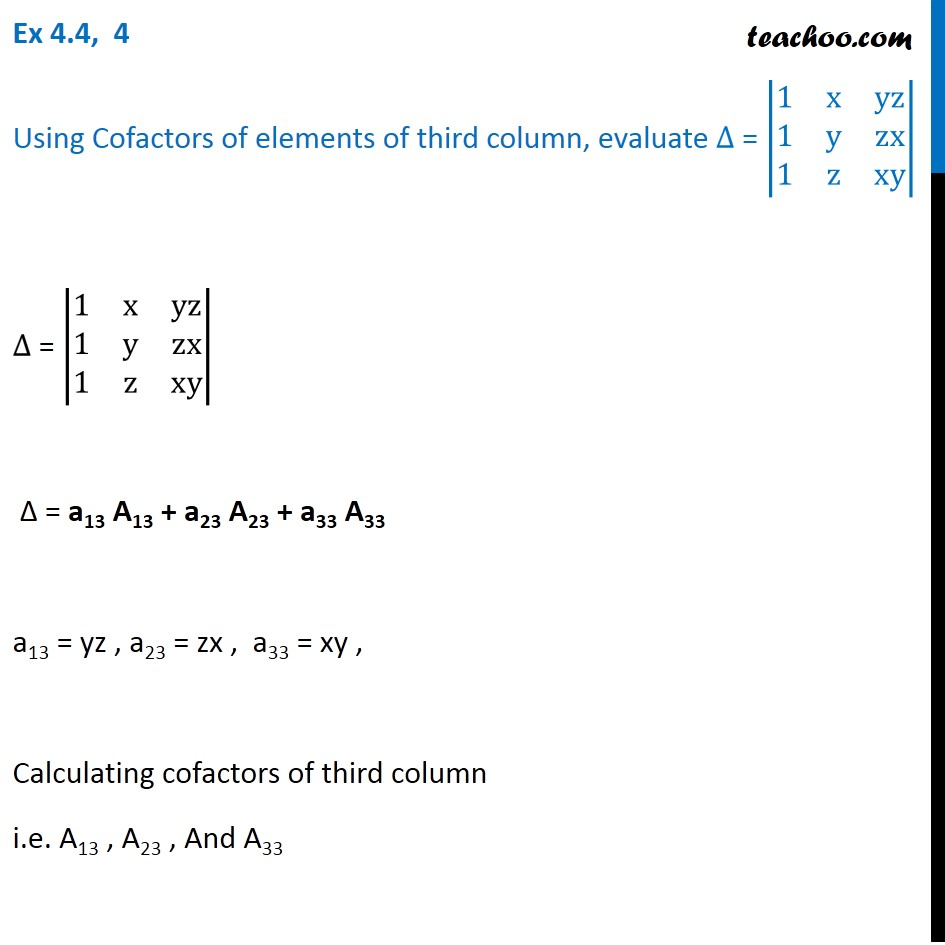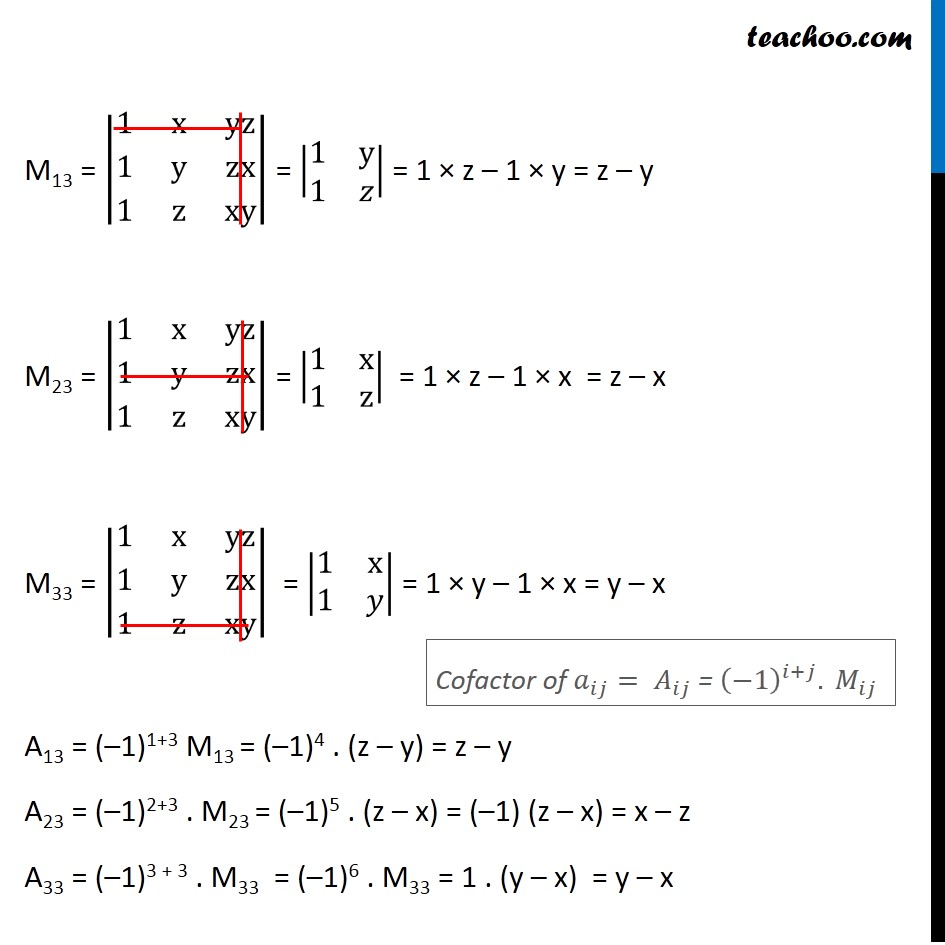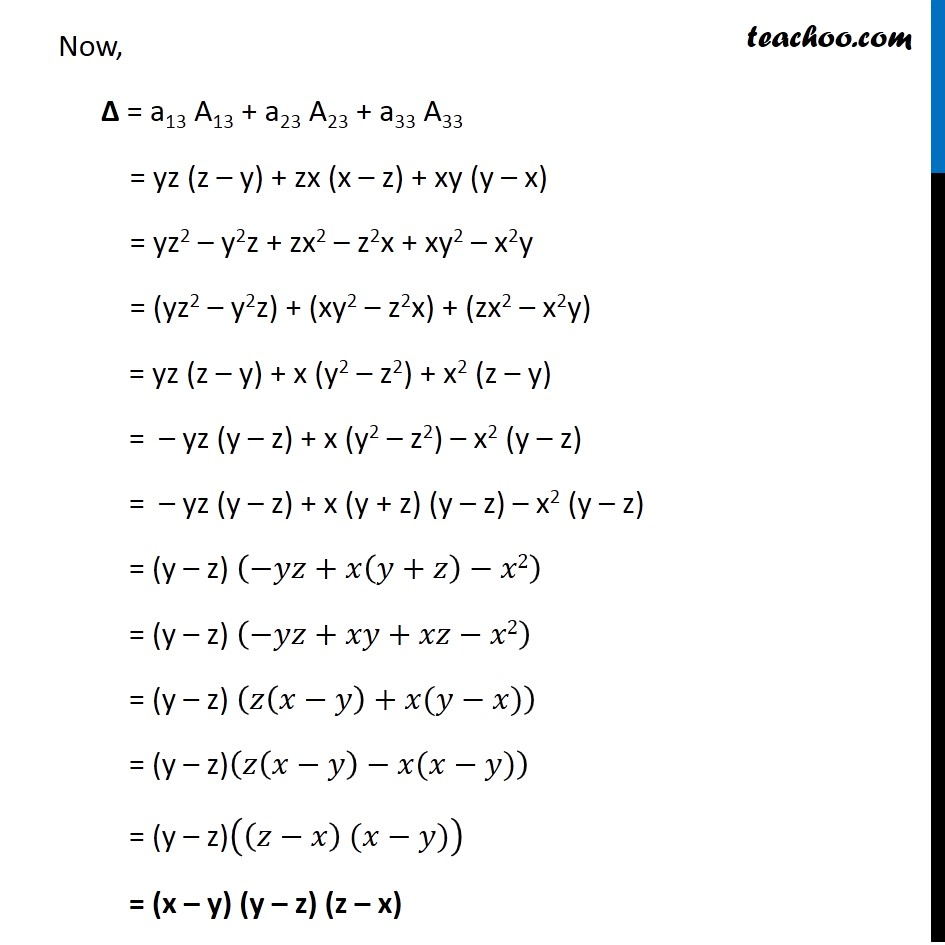Ex 4.4

Chapter 4 Class 12 Determinants
Serial order wiseSolve all your doubts with Teachoo Black (new monthly pack available now!)

### Transcript

Ex 4.4, 4 Using Cofactors of elements of third column, evaluate ∆ = |■8(1&x&yz@1&y&zx@1&z&xy)| ∆ = |■8(1&x&yz@1&y&zx@1&z&xy)| ∆ = a13 A13 + a23 A23 + a33 A33 a13 = yz , a23 = zx , a33 = xy , Calculating cofactors of third column i.e. A13 , A23 , And A33 M13 = |■8(1&x&yz@1&y&zx@1&z&xy)| = |■8(1&y@1&𝑧)| = 1 × z – 1 × y = z – y M23 = |■8(1&x&yz@1&y&zx@1&z&xy)| = |■8(1&x@1&z)| = 1 × z – 1 × x = z – x M33 = |■8(1&x&yz@1&y&zx@1&z&xy)| = |■8(1&x@1&𝑦)| = 1 × y – 1 × x = y – x A13 = (–1)1+3 M13 = (–1)4 . (z – y) = z – y A23 = (–1)2+3 . M23 = (–1)5 . (z – x) = (–1) (z – x) = x – z A33 = (–1)3 + 3 . M33 = (–1)6 . M33 = 1 . (y – x) = y – x Cofactor of 𝑎_𝑖𝑗= 𝐴_𝑖𝑗 = (−1)^(𝑖+𝑗). 𝑀_𝑖𝑗 Now, ∆ = a13 A13 + a23 A23 + a33 A33 = yz (z – y) + zx (x – z) + xy (y – x) = yz2 – y2z + zx2 – z2x + xy2 – x2y = (yz2 – y2z) + (xy2 – z2x) + (zx2 – x2y) = yz (z – y) + x (y2 – z2) + x2 (z – y) = – yz (y – z) + x (y2 – z2) – x2 (y – z) = – yz (y – z) + x (y + z) (y – z) – x2 (y – z) = (y – z) (−𝑦𝑧+𝑥(𝑦+𝑧)−𝑥2) = (y – z) (−𝑦𝑧+𝑥𝑦+𝑥𝑧−𝑥2) = (y – z) (𝑧(𝑥−𝑦)+𝑥(𝑦−𝑥)) = (y – z)(𝑧(𝑥−𝑦)−𝑥(𝑥−𝑦)) = (y – z)((𝑧−𝑥) (𝑥−𝑦)) = (x – y) (y – z) (z – x)